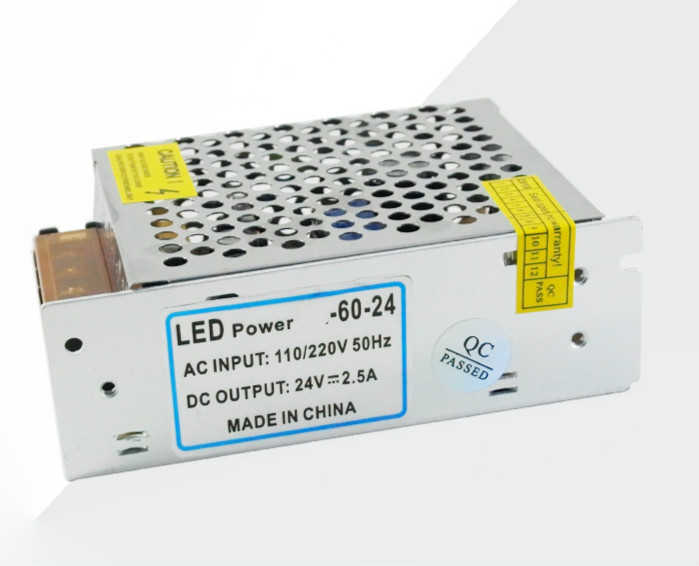EN
Language

## NEWS

What does constant voltage constant current power supply mean? Constant voltage constant current switching power supply is constant voltage or constant current?
2019-10-12 17:21:13
What does constant voltage constant current power supply mean? Constant voltage constant current switching power supply is constant voltage or constant current?
What is the meaning of constant voltage and current power source? What are the main USES of constant voltage and current power sources? With the development of social science and technology and the popularization of electrical equipment, constant voltage and constant current power supply has become more and more widely used in various fields and gradually becomes an indispensable power source. But is constant voltage and constant current power source constant voltage or constant current?
What does constant voltage constant current power supply mean?
The constant-voltage constant-current power supply is actually a switching power supply, but it has two control units, constant-voltage and constant-current, and two working states. When it is in the constant-voltage state, it works according to the characteristics of the constant-voltage power supply, and when it is in the constant-current state, it works according to the characteristics of the constant-current power supply.
Constant voltage constant current power supply is constant voltage or constant current?
To sum up, it can be seen that the constant-voltage constant-current power source is both constant-voltage and constant-current. When meet load resistance by constant pressure constant current power supply for a certain value, the power supply is in constant pressure constant current critical point (that is, when the voltage and current is the maximum value), with the increase of load resistance, the power within the constant pressure control unit start working, the output voltage remains the same, the current changes with the change of the load resistance. When the load resistance decreases and is less than the critical value, the constant current control unit in the power supply starts to work. At this time, the output current can remain unchanged and the voltage changes with the change of the load resistance.
Constant voltage constant current power supply characteristics
Constant voltage constant current circuit will not overload, short circuit and other phenomena, low power consumption, high efficiency, small size, light weight, convenient control, more applicable scope.
Internal components of constant voltage and current power supply
Constant voltage constant current power supply application
Constant-voltage constant-current power supply can be widely used in electric power, industrial control, communication, scientific research, battery charging, surface treatment, electrochemical, new energy, capacitors, motors, sewage treatment, electronic product production and testing, LED lighting, medical equipment, semiconductor equipment and other industries.